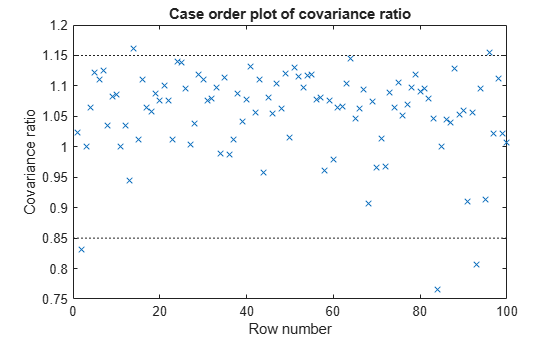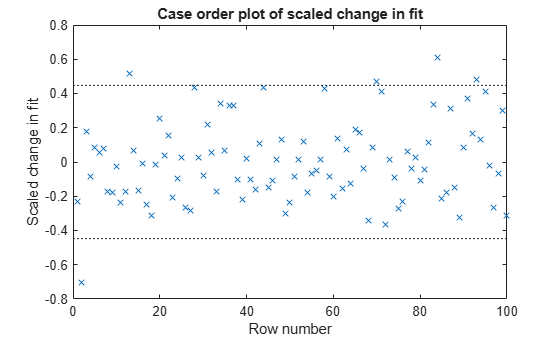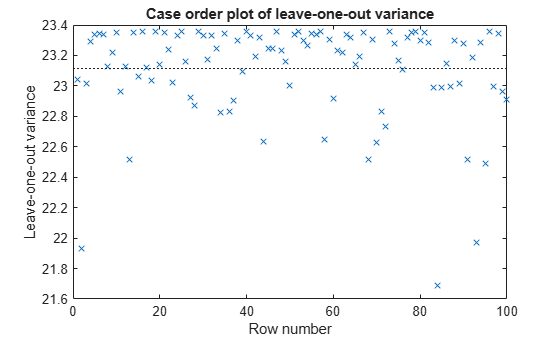## Delete-1 Statistics

### Delete-1 Change in Covariance (covratio)

#### Purpose

Delete-1 change in covariance (`covratio`) identifies the observations that are influential in the regression fit. An influential observation is one where its exclusion from the model might significantly alter the regression function. Values of covratio larger than 1 + 3*p/n or smaller than 1 – 3*p/n indicate influential points, where p is the number of regression coefficients, and n is the number of observations.

#### Definition

The covratio statistic is the ratio of the determinant of the coefficient covariance matrix with observation i deleted to the determinant of the covariance matrix for the full model:

`$\mathrm{cov}ratio=\frac{\mathrm{det}\left\{MSE\left(i\right){\left[{X}^{\prime }\left(i\right)X\left(i\right)\right]}^{-1}\right\}}{\mathrm{det}\left[MSE{\left({X}^{\prime }X\right)}^{-1}\right]}.$`

`CovRatio` is an n-by-1 vector in the `Diagnostics` table of the fitted `LinearModel` object. Each element is the ratio of the generalized variance of the estimated coefficients when the corresponding element is deleted to the generalized variance of the coefficients using all the data.

#### How To

After obtaining a fitted model, say, `mdl`, using `fitlm` or `stepwiselm`, you can:

• Display the `CovRatio` by indexing into the property using dot notation

`mdl.Diagnostics.CovRatio`

• Plot the delete-1 change in covariance using

`plotDiagnostics(mdl,'CovRatio')`
For details, see the `plotDiagnostics` method of the `LinearModel` class.

#### Determine Influential Observations Using CovRatio

This example shows how to use the `CovRatio` statistics to determine the influential points in data. Load the sample data and define the response and predictor variables.

```load hospital y = hospital.BloodPressure(:,1); X = double(hospital(:,2:5));```

Fit a linear regression model.

`mdl = fitlm(X,y);`

Plot the `CovRatio` statistics.

`plotDiagnostics(mdl,'CovRatio')`For this example, the threshold limits are 1 + 3*5/100 = 1.15 and 1 - 3*5/100 = 0.85. There are a few points beyond the limits, which might be influential points.

Find the observations that are beyond the limits.

`find((mdl.Diagnostics.CovRatio)>1.15|(mdl.Diagnostics.CovRatio)<0.85)`
```ans = 5×1 2 14 84 93 96 ```

### Delete-1 Scaled Difference in Coefficient Estimates (Dfbetas)

#### Purpose

The sign of a delete-1 scaled difference in coefficient estimate (Dfbetas) for coefficient j and observation i indicates whether that observation causes an increase or decrease in the estimate of the regression coefficient. The absolute value of a Dfbetas indicates the magnitude of the difference relative to the estimated standard deviation of the regression coefficient. A Dfbetas value larger than 3/sqrt(n) in absolute value indicates that the observation has a large influence on the corresponding coefficient.

#### Definition

Dfbetas for coefficient j and observation i is the ratio of the difference in the estimate of coefficient j using all observations and the one obtained by removing observation i, and the standard error of the coefficient estimate obtained by removing observation i. The Dfbetas for coefficient j and observation i is

`$Dfbeta{s}_{ij}=\frac{{b}_{j}-{b}_{j\left(i\right)}}{\sqrt{MS{E}_{\left(i\right)}}\left(1-{h}_{ii}\right)},$`

where bj is the estimate for coefficient j, bj(i) is the estimate for coefficient j by removing observation i, MSE(i) is the mean squared error of the regression fit by removing observation i, and hii is the leverage value for observation i. `Dfbetas` is an n-by-p matrix in the `Diagnostics` table of the fitted `LinearModel` object. Each cell of `Dfbetas` corresponds to the Dfbetas value for the corresponding coefficient obtained by removing the corresponding observation.

#### How To

After obtaining a fitted model, say, `mdl`, using `fitlm` or `stepwiselm`, you can obtain the Dfbetas values as an n-by-p matrix by indexing into the property using dot notation,

`mdl.Diagnostics.Dfbetas`

#### Determine Observations Influential on Coefficients Using `Dfbetas`

This example shows how to determine the observations that have large influence on coefficients using `Dfbetas`. Load the sample data and define the response and independent variables.

```load hospital y = hospital.BloodPressure(:,1); X = double(hospital(:,2:5));```

Fit a linear regression model.

`mdl = fitlm(X,y);`

Find the `Dfbetas` values that are high in absolute value.

```[row,col] = find(abs(mdl.Diagnostics.Dfbetas)>3/sqrt(100)); disp([row col])```
``` 2 1 28 1 84 1 93 1 2 2 13 3 84 3 2 4 84 4 ```

### Delete-1 Scaled Change in Fitted Values (Dffits)

#### Purpose

The delete-1 scaled change in fitted values (Dffits) show the influence of each observation on the fitted response values. Dffits values with an absolute value larger than 2*sqrt(p/n) might be influential.

#### Definition

Dffits for observation i is

`$Dffit{s}_{i}=s{r}_{i}\sqrt{\frac{{h}_{ii}}{1-{h}_{ii}}},$`

where sri is the studentized residual, and hii is the leverage value of the fitted `LinearModel` object. `Dffits` is an n-by-1 column vector in the `Diagnostics` table of the fitted `LinearModel` object. Each element in `Dffits` is the change in the fitted value caused by deleting the corresponding observation and scaling by the standard error.

#### How To

After obtaining a fitted model, say, `mdl`, using `fitlm` or `stepwiselm`, you can:

• Display the `Dffits` values by indexing into the property using dot notation

`mdl.Diagnostics.Dffits`

• Plot the delete-1 scaled change in fitted values using

`plotDiagnostics(mdl,'Dffits')`
For details, see the `plotDiagnostics` method of the `LinearModel` class for details.

#### Determine Observations Influential on Fitted Response Using `Dffits`

This example shows how to determine the observations that are influential on the fitted response values using `Dffits` values. Load the sample data and define the response and independent variables.

```load hospital y = hospital.BloodPressure(:,1); X = double(hospital(:,2:5));```

Fit a linear regression model.

`mdl = fitlm(X,y);`

Plot the `Dffits` values.

`plotDiagnostics(mdl,'Dffits')`The influential threshold limit for the absolute value of `Dffits` in this example is 2*sqrt(5/100) = 0.45. Again, there are some observations with `Dffits` values beyond the recommended limits.

Find the `Dffits` values that are large in absolute value.

`find(abs(mdl.Diagnostics.Dffits)>2*sqrt(4/100))`
```ans = 10×1 2 13 28 44 58 70 71 84 93 95 ```

### Delete-1 Variance (`S2_i`)

#### Purpose

The delete-1 variance (`S2_i`) shows how the mean squared error changes when an observation is removed from the data set. You can compare the `S2_i` values with the value of the mean squared error.

#### Definition

`S2_i` is a set of residual variance estimates obtained by deleting each observation in turn. The `S2_i` value for observation i is

`$S2_i=MS{E}_{\left(i\right)}=\frac{\sum _{j\ne i}^{n}{\left[{y}_{j}-{\stackrel{^}{y}}_{j\left(i\right)}\right]}^{2}}{n-p-1},$`

where yj is the jth observed response value. `S2_i` is an n-by-1 vector in the `Diagnostics` table of the fitted `LinearModel` object. Each element in `S2_i` is the mean squared error of the regression obtained by deleting that observation.

#### How To

After obtaining a fitted model, say, `mdl`, using `fitlm` or `stepwiselm`, you can:

• Display the `S2_i` vector by indexing into the property using dot notation

`mdl.Diagnostics.S2_i`

• Plot the delete-1 variance values using

`plotDiagnostics(mdl,'S2_i')`
For details, see the `plotDiagnostics` method of the `LinearModel` class.

#### Compute and Examine Delete-1 Variance Values

This example shows how to compute and plot S2_i values to examine the change in the mean squared error when an observation is removed from the data. Load the sample data and define the response and independent variables.

```load hospital y = hospital.BloodPressure(:,1); X = double(hospital(:,2:5));```

Fit a linear regression model.

`mdl = fitlm(X,y);`

Display the MSE value for the model.

`mdl.MSE`
```ans = 23.1140 ```

Plot the S2_i values.

`plotDiagnostics(mdl,'S2_i')`This plot makes it easy to compare the S2_i values to the MSE value of 23.114, indicated by the horizontal dashed lines. You can see how deleting one observation changes the error variance.